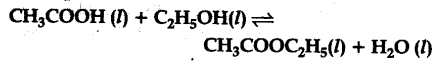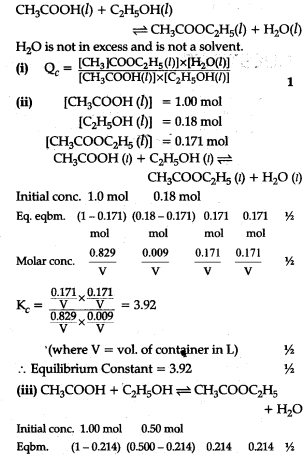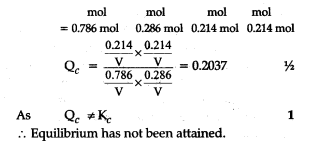# Ethyl acetate is formed by the reaction between ethanol and acetic acid and equilibrium is represented as:(i) Write the concentration ratio (reaction quo¬tient) Q., for this reaction (Note: water is not in excess and is not a solvent in this reaction).
(ii) At 293 K, if one starts with 1.00 ml of acetic acid and 0.18 mol of ethanol, there is 0.171 mol of ethyl acetate in the final equilibrium mixture. Calculate the equilibrium constant (iii) Starting with 0.5 mol of enthanol and 1.0 mol of acetic acid and maintaining it at 293 K, 0.214 mol of ethyl acetate is found after sometime. Has equilibrium been reached ?

The given reaction is